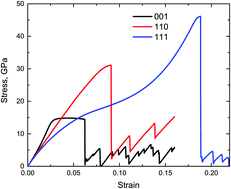## How to Calculate and Solve for Elongation Potential of Twin Deformation | Fracture MechanicsThe image above represents elongation potential of twin deformation.

To compute for elongation potential of twin deformation, two essential parameters are needed and these parameters are Distance between the 2 Slip Planes (S) and Angle of Deformation (x).

The formula for calculating elongation potential of twin deformation:

l’/l = (1 + Stanx)0.5

Where:

l’/l = Elongation Potential of Twin Deformation
S = Distance between the 2 Slip Planes
x = Angle of Deformation

Let’s solve an example;
Find the elongation potential of twin deformation when the distance between the 2 slip planes is 24 and the angle of deformation is 12°.

This implies that;

S = Distance between the 2 Slip Planes = 24
x = Angle of Deformation = 12°

l’/l = (1 + Stanx)0.5
l’/l = (1 + 24tan(12°))0.5
l’/l = (1 + 24(0.213))0.5
l’/l = (1 + 5.101)0.5
l’/l = (6.101)0.5
l’/l = 2.470

Therefore, the elongation potential of twin deformation is 2.470.